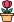엔지니어 게시판
LeetCode 솔루션 분류

# [11/29] 380. Insert Delete GetRandom O(1)

### 작성자 정보

•작성
• 작성일

• 170 조회
• 1 댓글
• 0 추천
• 0 비추천

### 본문

Medium

Implement the `RandomizedSet` class:

• `RandomizedSet()` Initializes the `RandomizedSet` object.
• `bool insert(int val)` Inserts an item `val` into the set if not present. Returns `true` if the item was not present, `false` otherwise.
• `bool remove(int val)` Removes an item `val` from the set if present. Returns `true` if the item was present, `false` otherwise.
• `int getRandom()` Returns a random element from the current set of elements (it's guaranteed that at least one element exists when this method is called). Each element must have the same probability of being returned.

You must implement the functions of the class such that each function works in average `O(1)` time complexity.

Example 1:

```Input
["RandomizedSet", "insert", "remove", "insert", "getRandom", "remove", "insert", "getRandom"]
[[], , , , [], , , []]
Output
[null, true, false, true, 2, true, false, 2]

Explanation
RandomizedSet randomizedSet = new RandomizedSet();
randomizedSet.insert(1); // Inserts 1 to the set. Returns true as 1 was inserted successfully.
randomizedSet.remove(2); // Returns false as 2 does not exist in the set.
randomizedSet.insert(2); // Inserts 2 to the set, returns true. Set now contains [1,2].
randomizedSet.getRandom(); // getRandom() should return either 1 or 2 randomly.
randomizedSet.remove(1); // Removes 1 from the set, returns true. Set now contains .
randomizedSet.insert(2); // 2 was already in the set, so return false.
randomizedSet.getRandom(); // Since 2 is the only number in the set, getRandom() will always return 2.
```

Constraints:

• `-231 <= val <= 231 - 1`
• At most `2 * ``105` calls will be made to `insert``remove`, and `getRandom`.
• There will be at least one element in the data structure when `getRandom` is called.
Accepted
584,547
Submissions
1,107,396

댓글 1

## 학부유학생님의 댓글

• 익명
• 작성일
Runtime: 414 ms, faster than 94.61% of Python3 online submissions for Insert Delete GetRandom O(1).
Memory Usage: 61.7 MB, less than 14.96% of Python3 online submissions for Insert Delete GetRandom O(1).
``````import random
class RandomizedSet:

def __init__(self):
self.num_list = []
self.num_map = {}
def insert(self, val: int) -> bool:
res = val not in self.num_map

if val not in self.num_map:
self.num_map[val] = len(self.num_list)
self.num_list.append(val)

return res

def remove(self, val: int) -> bool:
res = val in self.num_map
if val in self.num_map :
popidx = self.num_map[val]
self.num_list[popidx] = self.num_list[-1]
self.num_map[self.num_list[-1]] = popidx
self.num_list.pop()
del self.num_map[val]
return res

def getRandom(self) -> int:
return random.choice(self.num_list)

# Your RandomizedSet object will be instantiated and called as such:
# obj = RandomizedSet()
# param_1 = obj.insert(val)
# param_2 = obj.remove(val)
# param_3 = obj.getRandom()``````
전체 349 / 1 페이지

• 등록일 10:47
• 등록일 07:18
• 등록일 02:04
• 등록일 02:03
• 등록일 00:48
• 등록일 02.01
• 등록일 02.01
• 등록일 02.01
• 등록일 02.01
• 등록일 02.01
• 등록일 02.01
• 등록일 02.01

• 등록일 01.31
• 등록일 01.31
• 등록일 01.31
• 등록일 01.27
• 등록일 01.27
• 등록일 01.23
• 등록일 01.23

### Stats

• 현재 접속자 56 명
• 오늘 방문자 1,386 명
• 어제 방문자 1,716 명
• 최대 방문자 2,853 명
• 전체 회원수 536 명